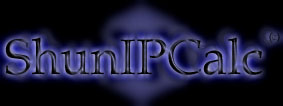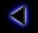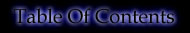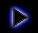.
How to convert binary to decimal ?
.
To convert binary to decimal, we start from the right to the left of the binary chain and at each bit, we associate the value 2^(character number), the first bit being bit number 0.
Note:
2^0 = 1
2^1 = 2
2^2 = 2 x 2 (2 times)
2^3 = 2 x 2 x 2 (3 times)
.
For example: 1 1 0 0 1 0 1 1
<------------------------------------------
power of 2: 2^7 2^6 2^5 2^4 2^3 2^2 2^1 2^0
Value: 128 64 32 16 8 4 2 1
.
The decimal value is the sum of the binary charaters times the powers of 2.
.
For the example above:
Result = 1 x 1 + 1 x 2 + 0 x 4 + 1 x 8 + 0 x 16 + 0 x 32 + 1 x 64 + 1 x 128 = 203
.
The minimum value is all bits set to "0" which is "0" in decimal and the maximum value for 8 bits (a byte) is all bits set to "1" which is "255" in decimal.
.
For a chain of n bits, the maximum is (2^n)-1. For example for a word (2 bytes), the maximum is 2^16-1=65535
.
..
Copyright (c) 2002 - Guillaume Péan
www.shunsoft.net